# Area Of A Hexagon

Here we will learn about the area of a hexagon.

There are also area worksheets based on Edexcel, AQA and OCR exam questions, along with further guidance on where to go next if you’re still stuck.

## What is the area of a hexagon?

The area of a hexagon is the amount of space inside a hexagon.

A hexagon is a 2D shape also known as a six-sided polygon.

### What is the area of a hexagon?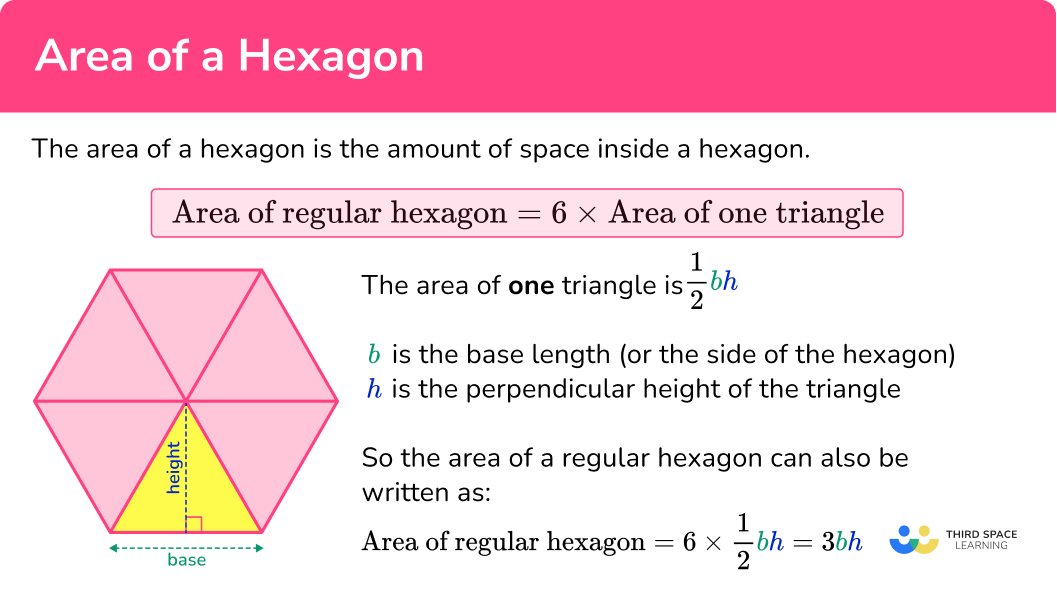### ● Area of an irregular hexagon

An irregular hexagon may have been formed by different shapes. The area of a hexagon can be calculated by working out the area of each of these shapes and adding them together.

For example,

Area of the irregular hexagon ABCDEF is

\text{total area }=(6\times 5)+(4\times 10)=30+40=70 \ cm^2.

### ● Area of a regular hexagon

A regular hexagon is a polygon that has 6 sides of equal length and 6 equal interior angles.

The area of a regular hexagon can be found by splitting the shape into congruent triangles. We can find the area of one of the triangles and then multiply by the number of sides to find the total area of the regular polygon.

This method will work for a regular hexagon, a regular octagon and so on. In the case of a regular hexagon the triangles will be equilateral triangles.

The area of a regular hexagon can be simplified into the formula

\text{area of regular hexagon }=6\times \text{area of one triangle}.

The area of the triangle can be found using the formula

A=\frac{1}{2}bh.

Where b is the base length (or the side of the hexagon) and h is the perpendicular height of the triangle. Sometimes these values will need to be calculated.

This means the area of a regular hexagon can also be written as

\text{area of regular hexagon }=6\times \frac{1}{2}bh=3bh.

### ● Angles in a regular hexagon

In order to work out the angles in a regular hexagon we can use the angle fact that states ‘the sum of angles at a point is 360^{\circ}’.

All 6 congruent triangles meet at the centre of the hexagon, forming a point, therefore all 6 angles around that centre point should sum to 360^{\circ}.

A regular hexagon can be split into 6 congruent equilateral triangles. So, the internal angles of an equilateral triangle are all 60^{\circ}.

The interior angles of these congruent triangles can be used in trigonometry to find the area of each of the equilateral triangles.

Two 60^{\circ} angles make one of the interior angles of a regular hexagon, so each of the 6 interior angles is 120^{\circ}.

Step-by-step guide: Equilateral triangles

Step-by-step guide: Area of an equilateral triangle

### ● Exact trigonometric values

Since the internal angles of equilateral triangles are 60^{\circ} and trigonometry may be used to calculate values, it is important to know the exact trigonometric values of 60^{\circ} for the purpose of any non-calculator questions.

\sin (60)=\frac{\sqrt{3}}{2}

\cos (60)=\frac{1}{2}

\tan (60)=\sqrt{3}

Step-by-step guide: Exact trig values

### ● The radii of a regular hexagon

In a regular hexagon the radius of the hexagon is equal to the length of the side.

The apothem of a regular polygon is always the radius of the inscribed circle, R_i.

It is also the minimum distance between any side of the polygon and its centre point.

When a regular hexagon is drawn within a circle the circle is known as the circumcircle and the radius is R_i.

The radius of the inscribed circle is perpendicular to the sides of the regular hexagon, meaning that it forms a right angle and meets the side at the midpoint.

### ● Area formula using the perimeter and apothem of a hexagon

As mentioned above, the height (or inscribed radius) in a regular hexagon is also known as the apothem of the hexagon.

There is another area of a regular hexagon formula that includes this

\text{area of regular hexagon}= \frac{1}{2}\times \text{perimeter}\times \text{apothem} .

The perimeter of the hexagon can be found by multiplying the length of one of the equal sides by 6.

You are not expected to know this formula at GCSE.

## How to find the area of a hexagon

In order to find the area of a hexagon:

1. Consider the hexagon as a compound shape and split it appropriately.
2. Calculate the area of the parts.
3. Calculate the total area of the hexagon.
4. Write the answer, including the correct units.

### Explain how find the area of a hexagon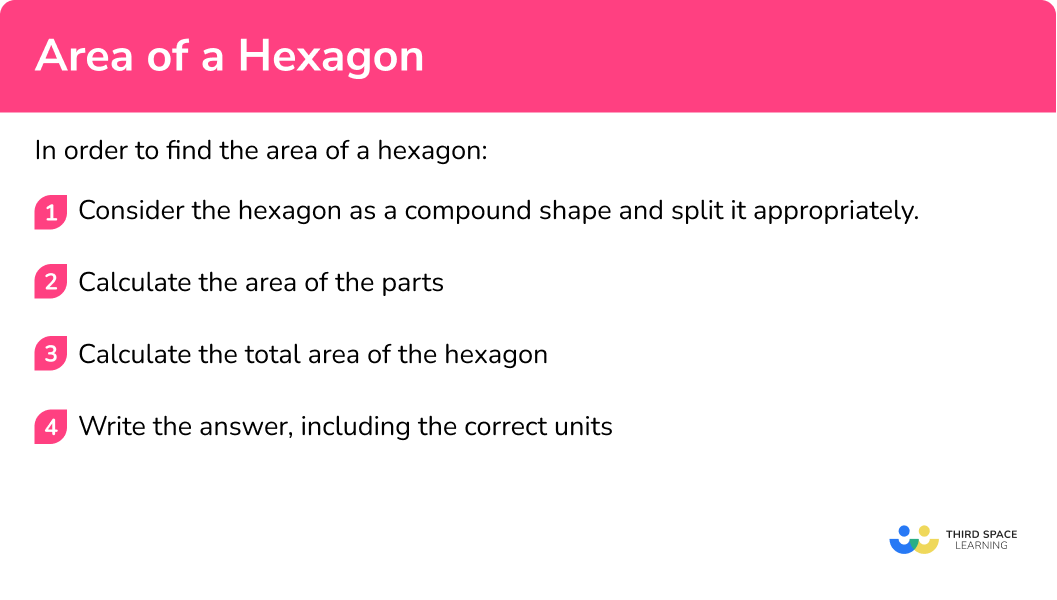## Related lessons on area

Area of a hexagon is part of our series of lessons to support revision on area. You may find it helpful to start with the main area lesson for a summary of what to expect, or use the step by step guides below for further detail on individual topics. Other lessons in this series include:

## Area of a hexagon examples

### Example 1: irregular hexagon

Calculate the area of the irregular hexagon ABCDEF.

1. Consider the hexagon as a compound shape and split it appropriately.

You can split the ‘L’ shape into two rectangles.

2Calculate the area of the parts.

The area of the top rectangle is

\text{area}=8\times (12-5)=56.

The area of the bottom rectangle is

\text{area}=5\times 13=65.

3Calculate the total area of the hexagon.

The total area of the hexagon is found by adding the area of the top and bottom rectangles together.

\text{Total Area}=65+56=121

4Write the answer, including the correct units.

The dimensions of the shape were given in centimetres, so the units of the area will be in square centimetres.

The area of hexagon ABCDEF is 121 \ cm^2.

### Example 2: irregular hexagon with inconsistent units

Calculate the area of the irregular hexagon ABCDEF.

You can split the ‘L’ shape into two rectangles.

The dimensions of the top rectangle are both in metres.

The area of the top rectangle is

\text{area}=0.5\times 1.2=0.6.

To calculate the area of the bottom rectangle we need to be consistent and also use metres. The area of the bottom rectangle is

\text{area}=0.9\times (0.8-0.5)=0.27.

The total area of the hexagon is found by adding the area of the two rectangles together.

\text{Total Area}=0.6+0.27=0.87

The dimensions of the shape used to calculate the area were metres, so the units of the area will be in square metres.

The area of hexagon ABCDEF is 0.87 \ m^2 (or 8700 \ cm^2 ).

### Example 3: area of a regular hexagon given the area of one of the equilateral triangles

Calculate the area of the regular hexagon ABCDEF given that the area of one of the congruent triangles is 14 \ cm^2.

The hexagon has already been split into six equilateral triangles for us.

The area of one triangle has been given in the question, 14 \ cm^2.

The area of the regular hexagon is

\begin{aligned} \text{area of regular hexagon}&=6\times \text{area of one triangle}\\\\ &=6\times 14\\\\ &=84 \end{aligned}

The area of hexagon ABCDEF is 84 \ cm^2.

### Example 4: area of a hexagon given the side length

Calculate the area of the regular hexagon ABCDEF given that the length of one side of the regular hexagon is 10 \ cm. Give your answer to 1 decimal place.

Here, the regular hexagon ABCDEF has been split into six congruent equilateral triangles.

There are three methods you can use to find the area of an equilateral triangle by forming a right triangle. The first two methods to find the height of the triangle are shown below.

The area of one of the equilateral triangles is

\begin{aligned} A&=\frac{1}{2}bh\\\\ &=\frac{1}{2}\times 10\times 5\sqrt{3}\\\\ &=25\sqrt{3} \end{aligned}

The third method uses the HIGHER trigonometry formula for the area of a triangle.

\begin{aligned} A&= \frac{1}{2}ab \sin C \\\\ &=\frac{1}{2} \times 10\times 10\times \sin (60)\\\\ &=25\sqrt{3} \end{aligned}

The area of the regular hexagon is

\begin{aligned} \text{area of regular hexagon}&=6\times \text{area of one triangle}\\\\ &=6\times 25\sqrt{3}\\\\ &=150\sqrt{3} \end{aligned}

The dimensions of the shape were given in centimetres, so the units of the area will be in square centimetres.

The area of hexagon ABCDEF is 259.8 \ cm^2 (to 1 decimal place).

### Example 5: area of a hexagon given the height (apothem)

Calculate the area of the regular hexagon ABCDEF given that the length of the apothem of the regular hexagon is 12 \ cm. Give your answer to 1 decimal place.

Here, the regular hexagon ABCDEF has been split into six congruent equilateral triangles.

You need to work out the base of the triangle.

This can be done using trigonometry with the angles inside the equilateral triangle.

x=12\div \tan(60)=4\sqrt{3}

The base of one of the equilateral triangles is

\text{base}=2x=2\times 4\sqrt{3}=8\sqrt{3}.

The area of one of the equilateral triangles is

\begin{aligned} A&=\frac{1}{2}bh\\\\ &=\frac{1}{2}\times 8\sqrt{3}\times 12\\\\ &=48\sqrt{3} \end{aligned}

The area of the regular hexagon is

\begin{aligned} \text{area of regular hexagon}&=6\times \text{area of one triangle}\\\\ &=6\times 48\sqrt{3}\\\\ &=288\sqrt{3} \end{aligned}

The dimensions of the shape were given in centimetres, so the units of the area will be in square centimetres.

The area of hexagon ABCDEF is 498.8 \ cm^2 (to 1 decimal place).

### Example 6: area of a hexagon given the radius of the circumcircle

Calculate the area of the regular hexagon ABCDEF given that the length from the centre of the hexagon to the vertices is 20 \ cm. Give your answer in exact form.

Here, the regular hexagon ABCDEF is split into six congruent equilateral triangles.

You need to work out the height of the triangle using trigonometry with the angles inside the equilateral triangle. The hypotenuse of the triangle is 20 \ cm.

\text{height}=\sin(60)\times 20=10\sqrt{3}

You need to work out the base of the triangle using trigonometry with the angles inside the equilateral triangle. The hypotenuse of the triangle is 20 \ cm.

x=\cos(60) \times 20=10

The base of one of the equilateral triangles is

\text{base}=2x=2\times 10=20.

The area of one of the equilateral triangles is

\begin{aligned} A&=\frac{1}{2}bh\\\\ &=\frac{1}{2}\times 20\times 10\sqrt{3}\\\\ &=100\sqrt{3} \end{aligned}

The alternative method uses the HIGHER trigonometry formula for the area of a triangle.

\begin{aligned} A&= \frac{1}{2}ab \sin C \\\\ &=\frac{1}{2} \times 20\times 20\times \sin (60)\\\\ &=100\sqrt{3} \end{aligned}

The area of the regular hexagon is

\begin{aligned} \text{area of regular hexagon}&=6\times \text{area of one triangle}\\\\ &=6\times 100\sqrt{3}\\\\ &=600\sqrt{3} \end{aligned}

The dimensions of the shape were given in centimetres, so the units of the area will be in square centimetres.

The area of hexagon ABCDEF is 600\sqrt{3} \ cm^2, in exact form.

### Common misconceptions

• Using the correct units

Remember that area is measured in square units. Square units include square centimetres (cm^2), square metres (m^2) and square millimetres (mm^2).

• Don’t round answers too early

It is important to not round decimals until the end of all the calculations. Rounding too early could result in an inaccurate answer.

• Take care of which dimensions you are using

It is important to make sure you keep track of which dimensions you have been given and which dimensions you are calculating. Take care with each step of your calculations. Make use of any given diagrams and mark on them as you work through the problem.

• Exact form

It is important to leave your answer in exact form if asked to. This may involve roots (for example, \sqrt{2} ) and/or \pi.

### Practice area of a hexagon questions

1. Calculate the area of the irregular hexagon ABCDEF. Give your answer in square centimetres.89 \ cm^2119 \ cm^232 \ cm^2123 \ cm^2The irregular hexagon ABCDEF can be split into two rectangles.The area of the top rectangle is

\text{Area}=13\times 5=65.

The area of the bottom rectangle is

\text{Area}=6\times (9-5)=24.

The total area of the irregular hexagon is found by adding the two separate areas together.

\text{Total area}=65+24=89 \ cm^2.

2. Calculate the area of the irregular hexagon ABCDEF. Give your answer in square metres.8.5 \ m^24.35 \ m^25.26 \ m^29.29 \ m^2The irregular hexagon ABCDEF can be split into two rectangles. The dimensions can also be converted into metres.

190 \ cm=1.9 \ m

220 \ cm=2.2 \ mThe area of the top rectangle is

\text{Area}=1.3\times 1.9=2.47.

The area of the bottom rectangle is

\text{Area}=3.1\times (2.2-1.3)=2.79.

The total area of the irregular hexagon is found by adding the two separate areas together.

\text{Total area}=2.47+2.79=5.26 \ m^2.

3. Calculate the area of the regular hexagon ABCDEF given that the area of one of the congruent triangles is 50 \ cm^2.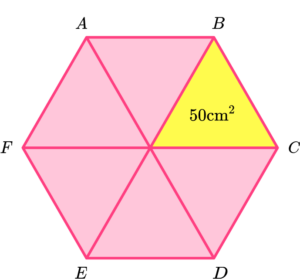200 \ cm^2250 \ cm^2600 \ cm^2300 \ cm^2The area of the regular hexagon is

\begin{aligned} \text{Area of regular hexagon}&=6\times \text{area of one triangle}\\\\ &=6\times 50\\\\ &=300 \ cm^2 \end{aligned}

4. Calculate the area of the regular hexagon ABCDEF given that the length of one side of the regular hexagon is 12 \ cm.372 \ cm^2374 \ cm^2312 \ cm^2216 \ cm^2The area of a regular hexagon can be found by splitting it into 6 equilateral triangles and finding the area of one of the triangles and multiplying it by 6.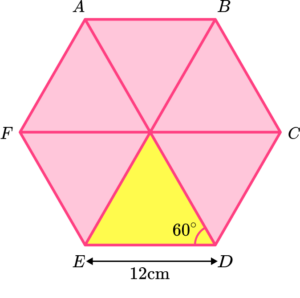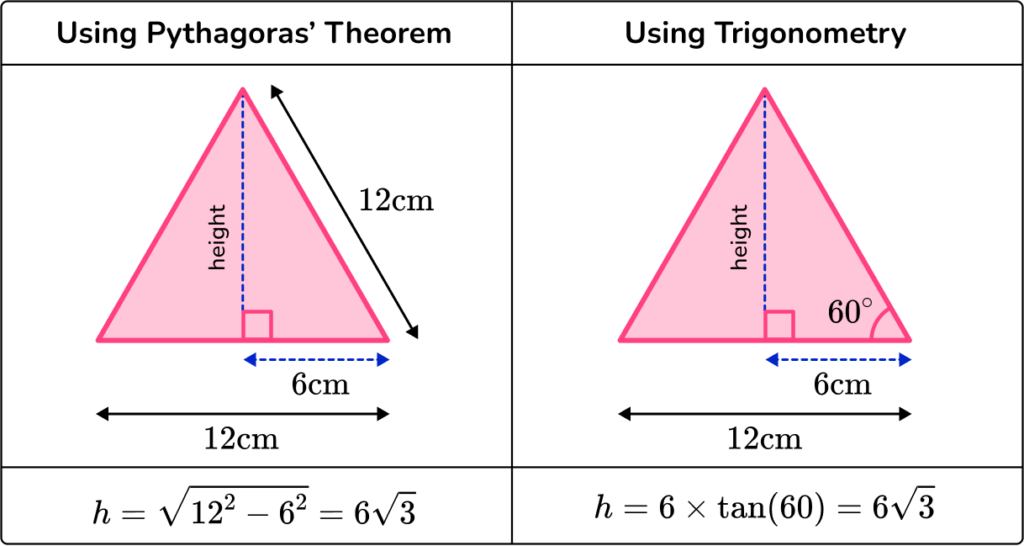The area of one of the equilateral triangles is

\begin{aligned} A&=\frac{1}{2}bh\\\\ &=\frac{1}{2}\times 12\times 6\sqrt{3}\\\\ &=36\sqrt{3} \end{aligned}

The third method uses the HIGHER trigonometry formula for the area of a triangle.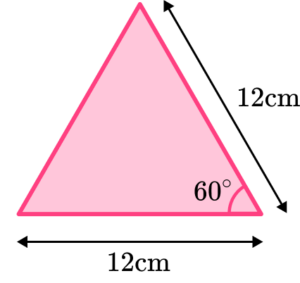\begin{aligned} A&= \frac{1}{2}ab \sin C \\\\ &=\frac{1}{2} \times 12\times 12\times \sin (60)\\\\ &=36\sqrt{3} \end{aligned}

The area of the regular hexagon is

\begin{aligned} \text{Area of regular hexagon}&=6\times \text{area of one triangle}\\\\ &=6\times 36\sqrt{3}\\\\ &=216\sqrt{3}\\\\ &=374 \ cm^2 \end{aligned}

5. Calculate the area of the regular hexagon ABCDEF given that the length of the apothem of the regular hexagon is 5 \ cm.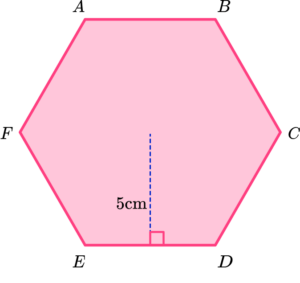15\sqrt{3} \ cm^230\sqrt{3} \ cm^250\sqrt{3} \ cm^245\sqrt{3} \ cm^2The area of a regular hexagon can be found by splitting it into 6 equilateral triangles and finding the area of one of the triangles and multiplying it by 6.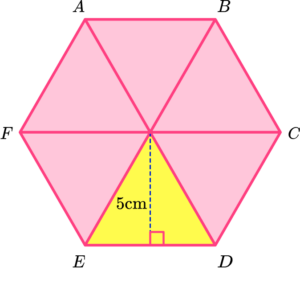The base of the triangle is calculated using trigonometry with the angles inside the equilateral triangle.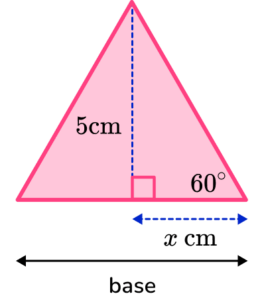x=5\div \tan(60)=\frac{5\sqrt{3}}{3}

The base of one of the equilateral triangles is

\text{base}=2x=2\times \frac{5\sqrt{3}}{3}=\frac{10\sqrt{3}}{3} .

The area of one of the equilateral triangles is

\begin{aligned} A&=\frac{1}{2}bh\\\\ &=\frac{1}{2}\times \frac{10\sqrt{3}}{3}\times 5\\\\ &=\frac{25\sqrt{3}}{3} \end{aligned}

The area of the regular hexagon is

\begin{aligned} \text{Area of regular hexagon}&=6\times \text{area of one triangle}\\\\ &=6\times \frac{10\sqrt{3}}{3}\\\\ &=50\sqrt{3} \end{aligned}

6. Calculate the area of the regular hexagon ABCDEF given that the length from the centre of the hexagon to the vertices is 6 \ cm.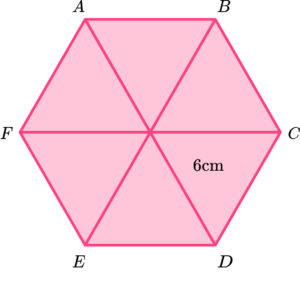15\sqrt{3} \ cm^212\sqrt{3} \ cm^218\sqrt{3} \ cm^254\sqrt{3} \ cm^2The area of a regular hexagon can be found by splitting it into 6 equilateral triangles and finding the area of one of the triangles and multiplying by 6.

The height of the triangle can be calculated using trigonometry and the angles in the equilateral triangle.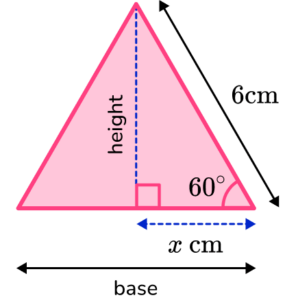\text{height}=\sin(60)\times 6=3\sqrt{3}

The base of the triangle can be calculated using trigonometry and the angles in the equilateral triangle.

x=\cos(60) \times 6=3

The base of one of the equilateral triangles is

\text{base}=2x=2\times 3=6.

The area of one of the equilateral triangles is

\begin{aligned} A&=\frac{1}{2}bh\\\\ &=\frac{1}{2}\times 6\times 3\sqrt{3}\\\\ &=9\sqrt{3} \end{aligned}

The alternative method uses the HIGHER trigonometry formula for the area of a triangle.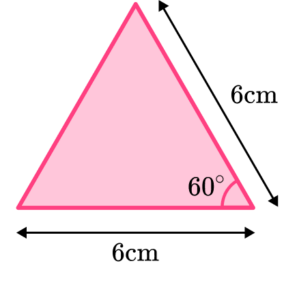\begin{aligned} A&= \frac{1}{2}ab \sin C \\\\ &=\frac{1}{2} \times 6\times 6\times \sin (60)\\\\ &=9\sqrt{3} \end{aligned}

The area of the regular hexagon is

\begin{aligned} \text{Area of regular hexagon}&=6\times \text{area of one triangle}\\\\ &=6\times 9\sqrt{3}\\\\ &=54\sqrt{3} \end{aligned}

### Area of a hexagon GCSE questions

1. The diagram shows the irregular hexagon ABCDEF. Calculate the area of the irregular hexagon. Give your answer in square centimetres.(3 marks)

12 \times 4=48

(1)

48+(5 \times 3)

(1)

63 \ cm^2

(1)

2. (a) The diagram shows a regular hexagon ABCDEF with side length 40 \ cm.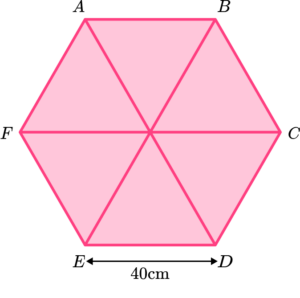Calculate the area of the regular hexagon ABCDEF.

(b) The hexagon is the base of a pyramid with height 80 \ cm.

Calculate the volume of the hexagonal-based pyramid.

(6 marks)

(a)

60^{\circ} \ or \ \frac{\sqrt{3}}{2} seen, may be on diagram.

(1)

Height = 20\sqrt{3}

(1)

400\sqrt{3}=692.820…

(1)

2400\sqrt{3}=4157 \ cm^2

(1)

(b)

\frac{1}{3} \times 2400\sqrt{3} \times 80

(1)

64000\sqrt{3}=110 \ 851 =111 \ 000 \ cm^3

(1)

3. The diagram shows a circle with radius  10 \ cm.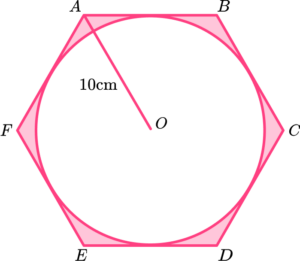ABCDEF is a regular hexagon.

Leave your answer as an exact value in the form a \pi -b\sqrt{3}.

(4 marks)

Area of circle is \pi \times 10^2=100 \pi .

(1)

Area of 1 triangle is 25\sqrt{3} .

(1)

Area of hexagon is 150\sqrt{3} .

(1)

Shaded area is 100 \pi-150\sqrt{3} .

(1)

## Learning checklist

You have now learned how to:

• Find the area of an irregular hexagon using compound areas
• Find the area of a regular polygon
• Solve problems involving the area of a regular polygon

## Still stuck?

Prepare your KS4 students for maths GCSEs success with Third Space Learning. Weekly online one to one GCSE maths revision lessons delivered by expert maths tutors.

Find out more about our GCSE maths tuition programme.